## Project Description

Problem Statement:

Implement Modified Butterfly Subdivision Surfacing on the CPU.

Background:

Subdivision surfaces are an increasingly popular way of creating realistic and high-fidelity model representations from a simpler control mesh. There are also two main kinds of subdivision: interpolating and approximating. Here, I would like to pursue implementing an interpolating subdivision method called Modified Butterfly.

General Algorithm:

1. Load OBJ mesh into an OpenMesh data structure
2. Iterate over the mesh and subdivide using Mod. Butterfly rules
3. Display subdivided mesh using OpenGL

Goals:

1. Implement Modified Butterfly Subdivisions on the CPU
2. Show a side-by-side comparison with the control mesh
3. Allow users to specify obj files and subdivision level at the command line

## Implementation

What the Butterfly Method?

Before we can implement anything we must first ask "What is this Modified Butterfly subdivision anyway?" Well, back in back in a day team came up with a way of treating all points of a triangulated mesh as the limit surface of subdivision (think of it like a "surface" Basis spline). This contrasts the Catmull-Clark subdivision where the control points of a mesh are not on the limit surface.

The Butterfly method only really worked on meshes where the vertices were of valence 5. This was because this method was based on an 8-point "butterfly" stencil as shown in Figure 1. Notice how the two vertices labeled "a" are connected to 5 other vertices.

What does this have to do with Modified Butterfly?

Modified Butterfly attempts to address the issues with the original Butterfly method (oscillating surface ripples, only valence 5 support) by adding rules for what are called "extraordinary points" (valence != 6) and modifying the original 8-point stencil to a 10-point stencil. The 10-point stencil is shown in Figure 2.

•Figure 1: 8-point stencil

•Figure 2: 10-point stencil

Here's what I gather the general algorithm is for Modified Butterfly:

``` 1> for each edge in mesh
2>     compute a new vertex based on edge endpoints
3>     add vertex to mesh (store in map:edge->vertex)
4> end:for
5> for each face in mesh
6>     remove face from mesh
7>     for each corner of face
8>         create face using corner and new vertices
9>          given by connecting edges (use map)
10>     end:for
11>     create face using new edge vertex (use map)
12> end:for```

Next we need to know about how to determine new vertices at each edge. A quick way to verify our previous algorithm is (generally) correct is to use the simplest scheme, which is just take the average of the endpoints of a given edge and make that the new vertex. Modified Butterfly uses four cases for weighting a new vertex:

1. Both Vertices are Valence 6: Compute the new point P on the edge between two the vertices by summing up the result of multiplying the weights a, b, c, and d (Figure 3) by the corresponding vertex on the 10-point stencil (Figure 2).
2. One Vertex is Valence 6, the Other is Extraordinary: Compute he new point P on the edge between the two vertices by summing up the result of multiplying the weights of vertices around the extraordinary point, then adding 3/4 the extraordinary point (see Figure 4). (NOTE: We don't do anything with the Valence 6 point)
3. Both Vertices are Extraordinary: Same as (2) but do so for both vertices and take the average.
4. One Vertex Lies on a Border: Zorin suggests using 4-point splines, but I did not implement this.
•Figure 3: Weighting scheme for pair of Valence 6 vertices.

•Figure 4: Weighting scheme for an extraordinary vertex. The weight "v" applies to the extraordinary vertex itself. Each ej is applied to the vertices in counterclockwise order starting with the vertex at the other end of the current edge (e0).

For my mesh representation I used OpenMesh. OpenMesh has a fairly decent interface for loading .obj files and then manipulating the resulting mesh.

## Results

The source code for this project can be found on Github.

In addition to implementing Modified Butterfly (surprisingly not that much code), I encountered many cool looking bugs while subdividing and applying Modified Btterfly weighting. This ranged from weird, to noisy, to unexpectedly beautiful limit surfaces.

1. ModButterfly: w = -1/16
•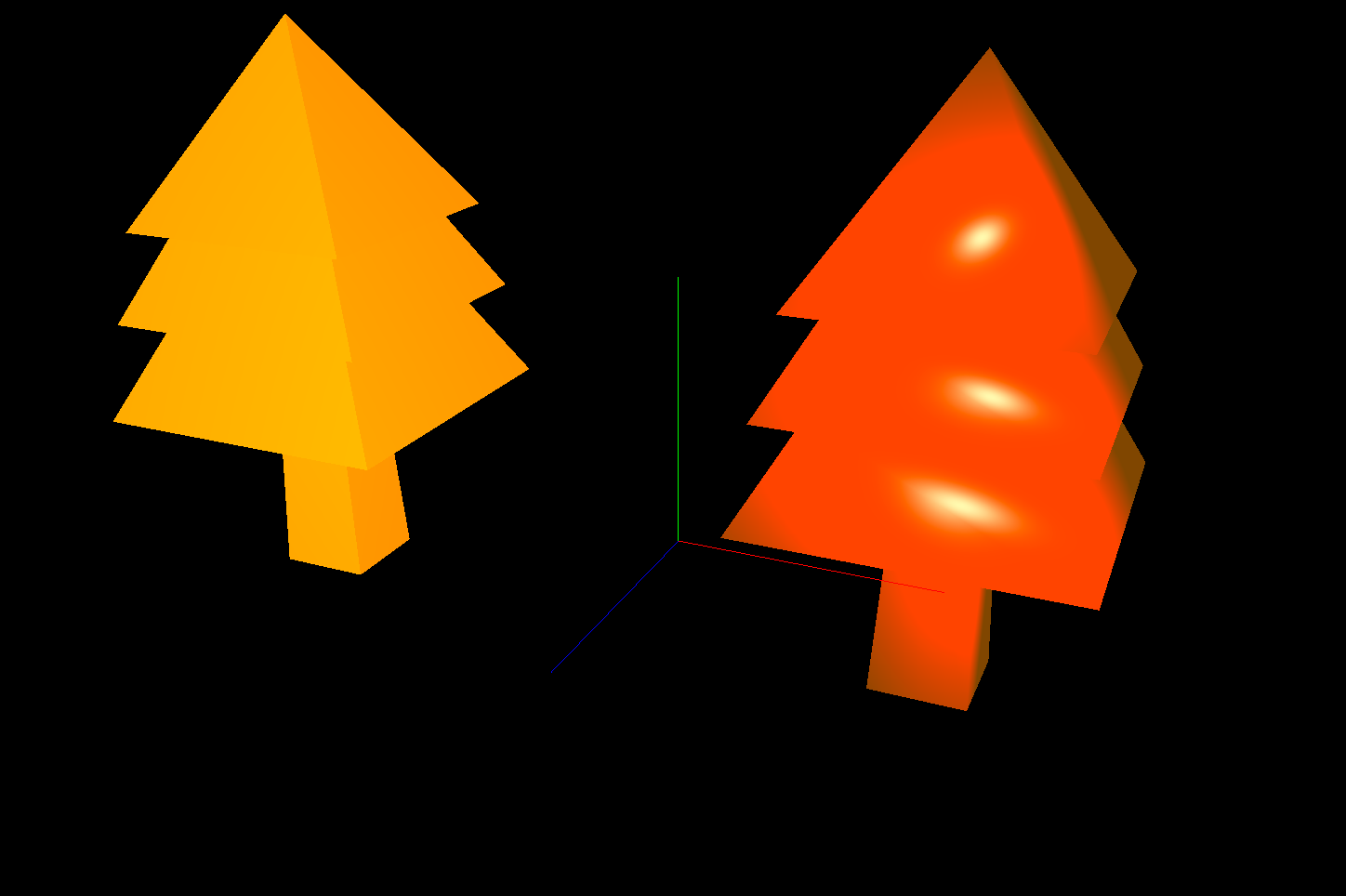Figure: Evergreen tree (no subdivision, filled)

•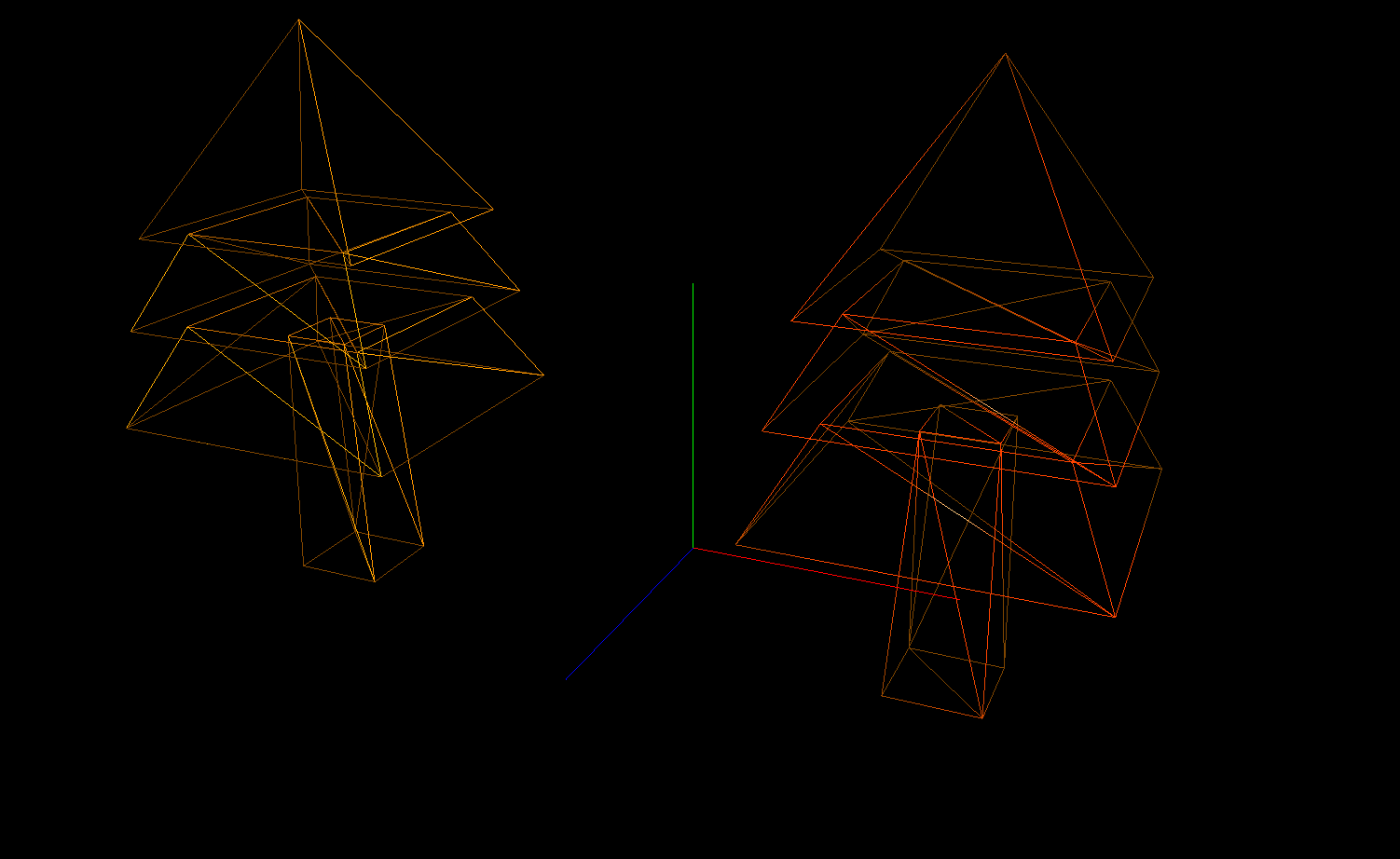Figure: Evergreen tree (no subdivision, lines)

•Figure: Evergreen tree (subdivision 1, filled)

•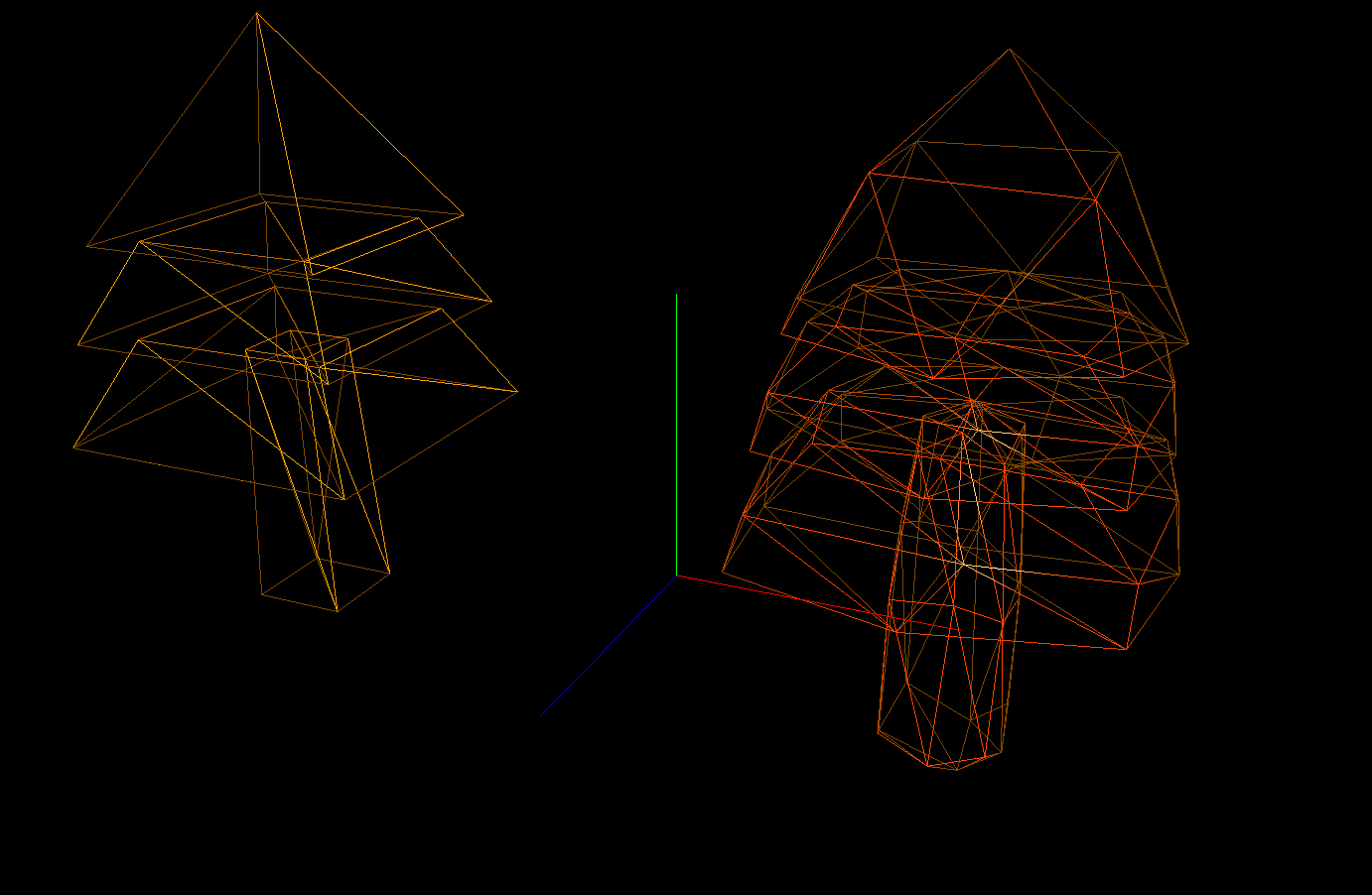Figure: Evergreen tree (subdivision 1, lines)

•Figure: Evergreen tree (subdivision 2, filled)

•Figure: Evergreen tree (subdivision 2, lines)

•Figure: Evergreen tree (subdivision 3, filled)

•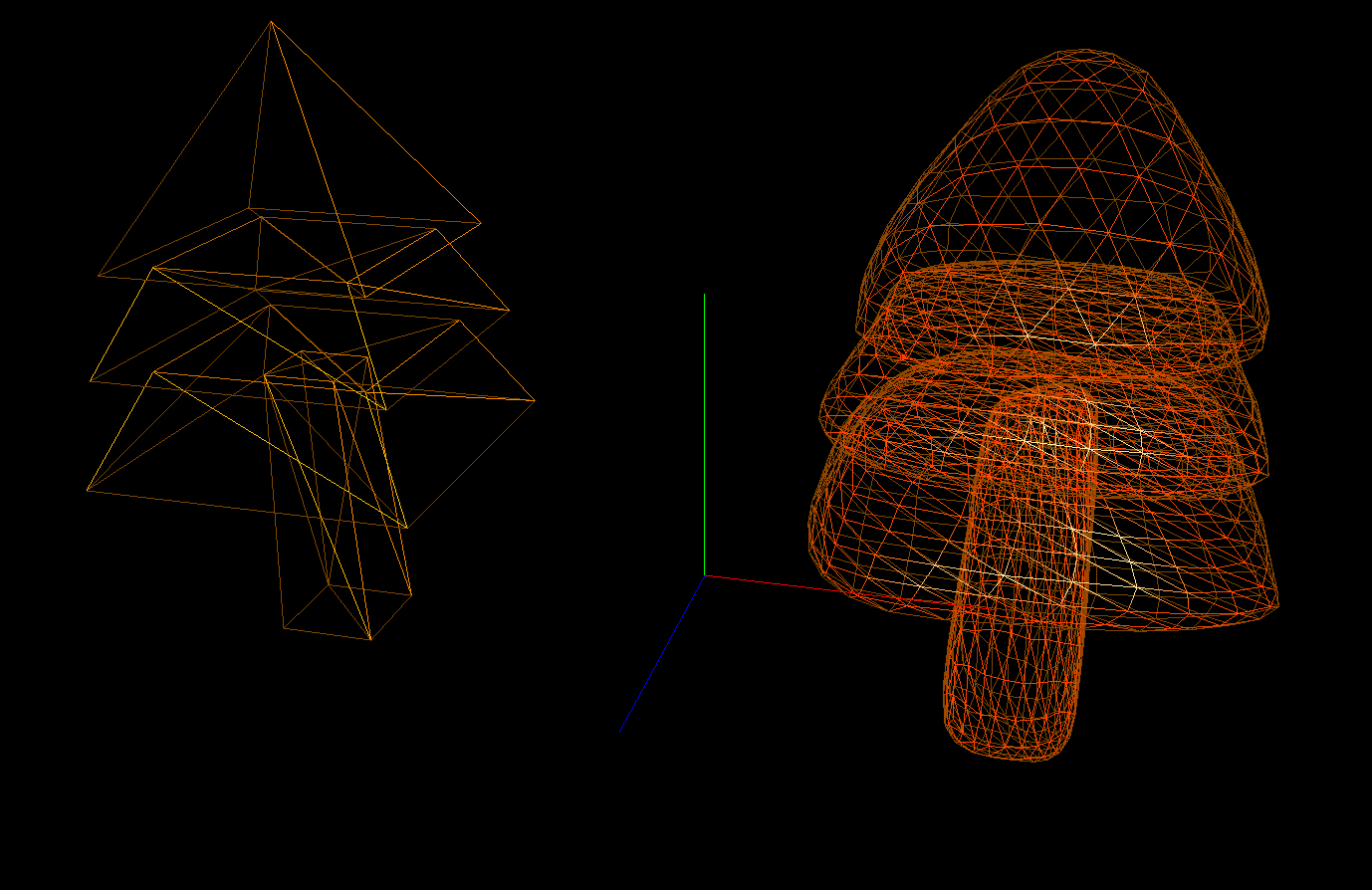Figure: Evergreen tree (subdivision 3, lines)

•Figure: Evergreen tree (subdivision 4, filled)

•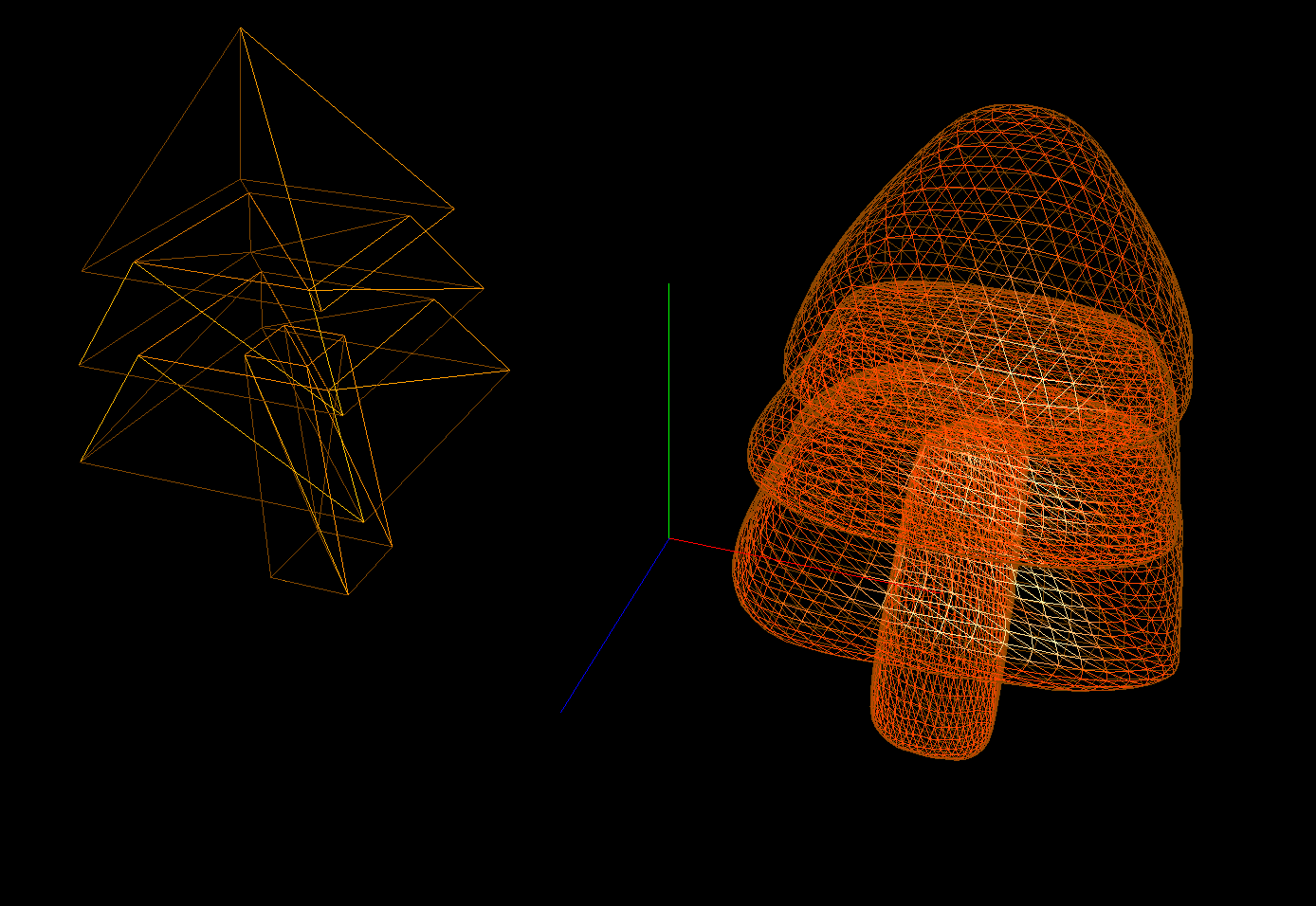Figure: Evergreen tree (subdivision 4, lines)

•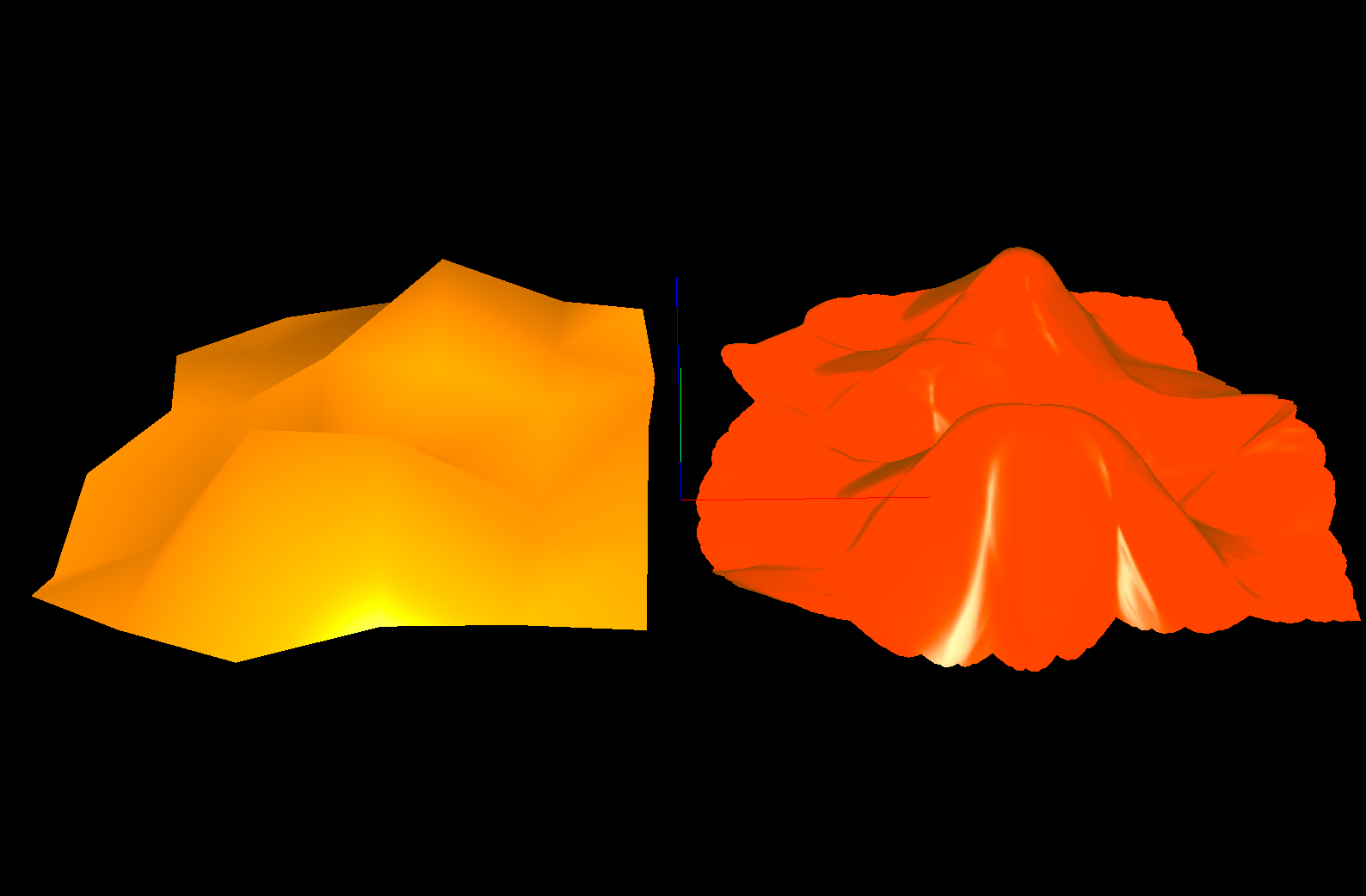Figure: Subdivided plane

•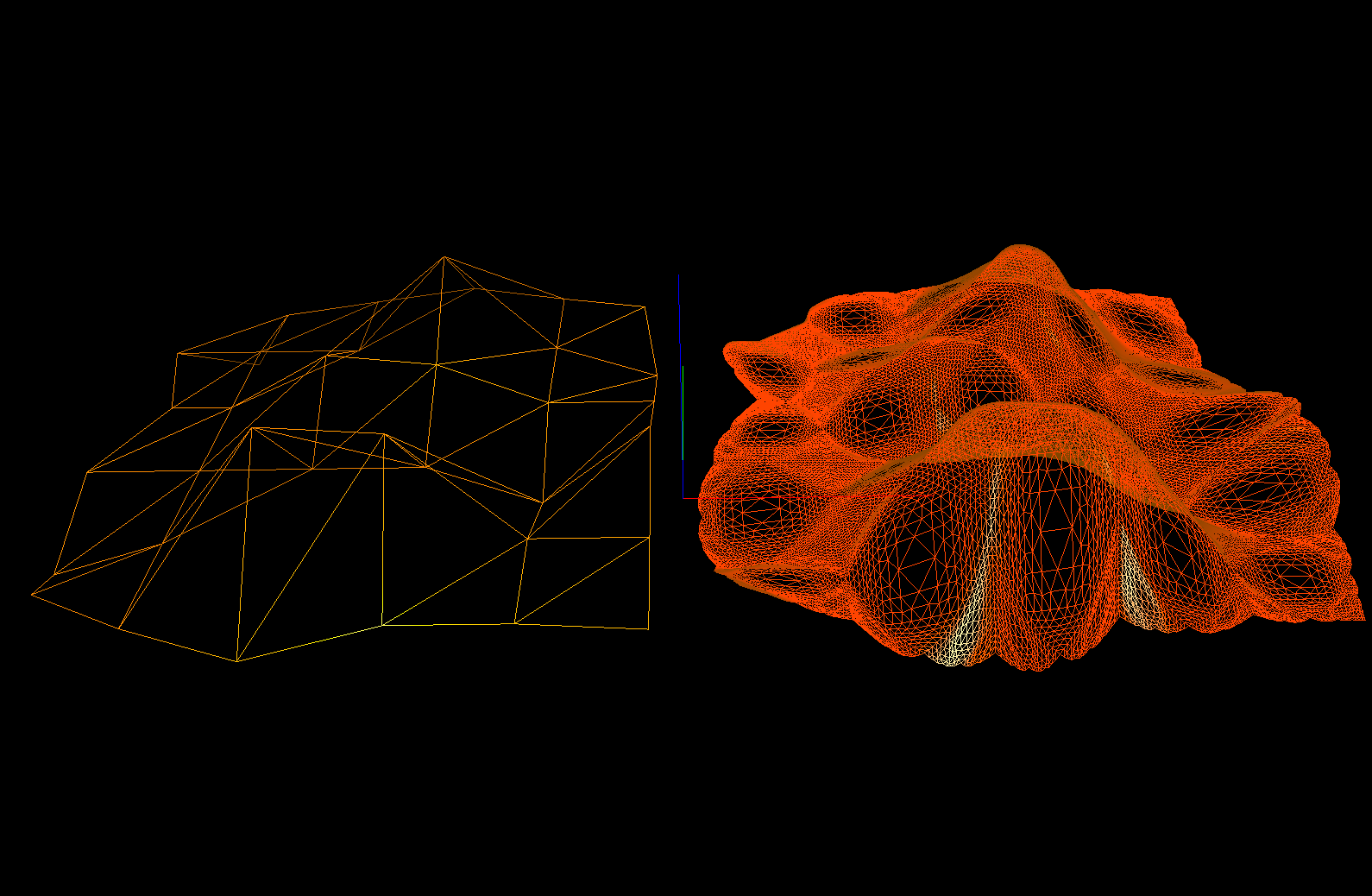Figure: Sudivided plane (lines)

2. ModButterflyRoses: w = -1/16, no averaging on rule #3
•Figure: A Cube that has been turned into a "rose".

•Figure: An evergreen has been turned into a "rose".

3. ModButterflySpikes:
•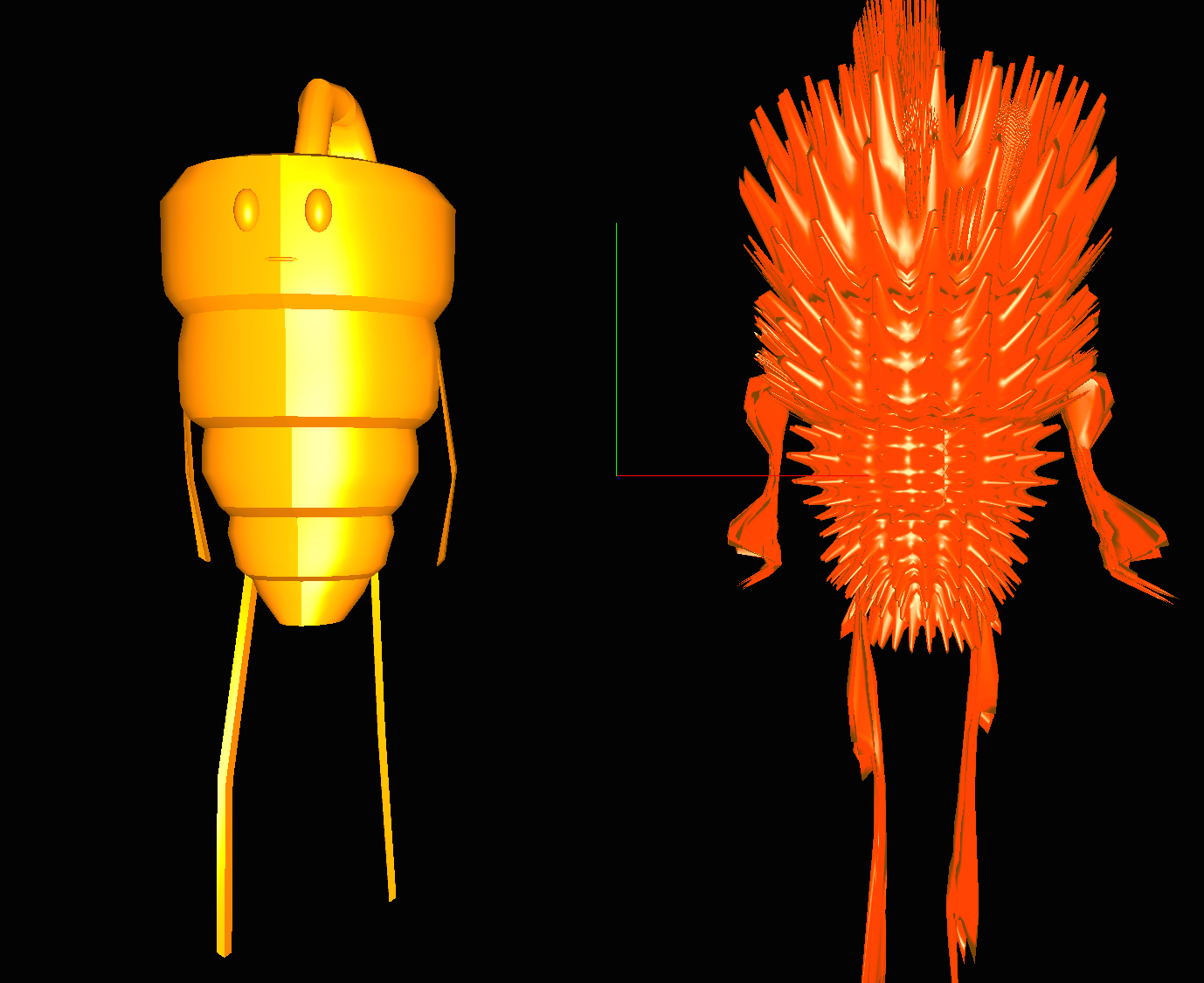Figure: A Carrot that has been spike-ified

4. SubdivTriforce
•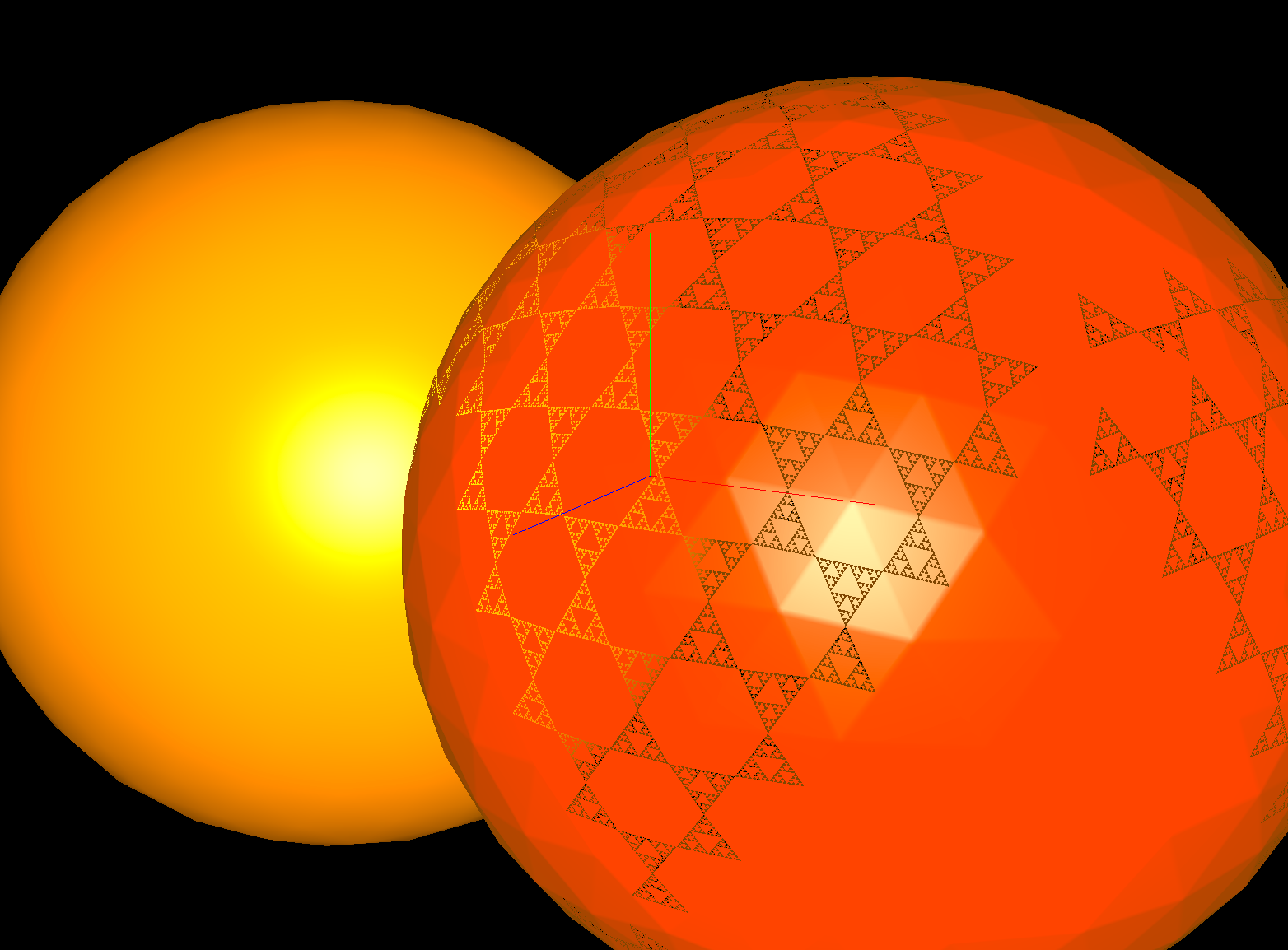Figure: A sphere that randomly lost triangles.

5. Other: I'm not entirely show how these hapenned, but they did :)
•Figure: A Cube that has been spike-ified

•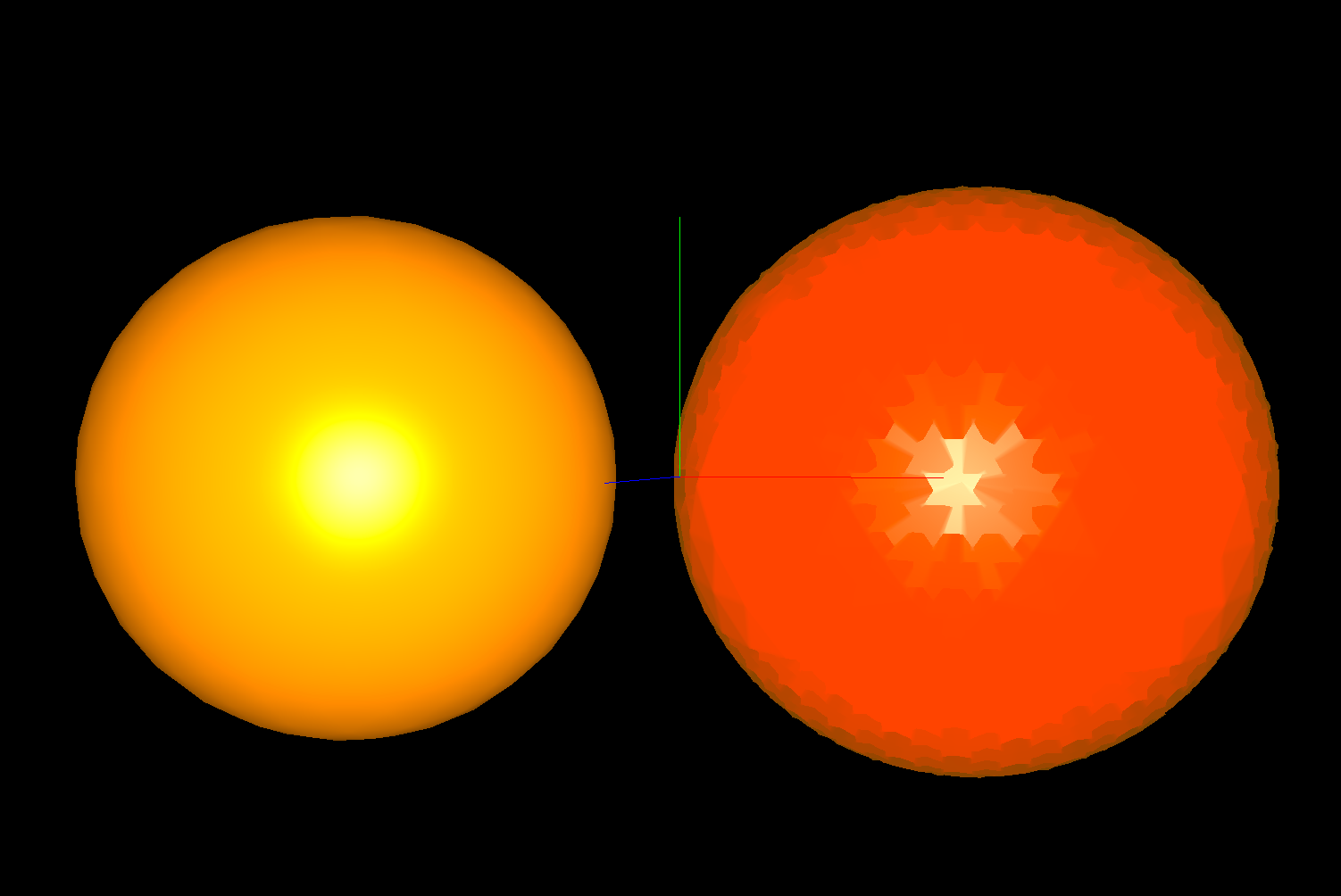Figure: A sphere that's kinda like a golf ball?

6. Video

• This video shows off the program I made.